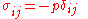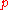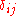xHydrostatic fluidEncyclopedia
In atmospheric dynamics and fluid dynamics
Fluid dynamics
In physics, fluid dynamics is a sub-discipline of fluid mechanics that deals with fluid flow—the natural science of fluids in motion. It has several subdisciplines itself, including aerodynamics and hydrodynamics...

, a hydrostatic fluid is a fluid in which fluid stresses act isotropically and fluid elements are in local equilibrium with one another. Thus, all directional fluid stresses, normally represented as vectors, can be summarized by a scalar quantity
Scalar (physics)
In physics, a scalar is a simple physical quantity that is not changed by coordinate system rotations or translations , or by Lorentz transformations or space-time translations . This is in contrast to a vector...

called the hydrostatic pressure, which can still depend on position.

The stress tensor takes on the form:,

where:is the hydrostatic pressure, andis the kronecker delta

The equilibrium which exists in a hydrostatic fluid is often the result of a balance between
forces that compress the fluid and forces that resist compression. For example, on earth, the atmosphere is compressed against the surface by gravity and supported by its internal energy
Internal energy
In thermodynamics, the internal energy is the total energy contained by a thermodynamic system. It is the energy needed to create the system, but excludes the energy to displace the system's surroundings, any energy associated with a move as a whole, or due to external force fields. Internal...

. This is often referred to as hydrostatic equilibrium
Hydrostatic equilibrium
Hydrostatic equilibrium or hydrostatic balance is the condition in fluid mechanics where a volume of a fluid is at rest or at constant velocity. This occurs when compression due to gravity is balanced by a pressure gradient force...

.